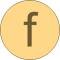# Gibbs2_ph

Function to calculate analytic derivatives for computing T given p and h# Syntax

nderivs = Gibbs2_ph(g)

# Inputs (1)

g Type: GibbsDerivs2 Description: Dimensionless derivatives of Gibbs function

# Outputs (1)

nderivs Type: NewtonDerivatives_ph Description: Derivatives for Newton iteration to calculate d and t from p and h